Courses

# Points to Remember- Linear Equations in One Variable Class 8 Notes | EduRev

## Mathematics (Maths) Class 8

Created by: Full Circle

## Class 8 : Points to Remember- Linear Equations in One Variable Class 8 Notes | EduRev

The document Points to Remember- Linear Equations in One Variable Class 8 Notes | EduRev is a part of the Class 8 Course Mathematics (Maths) Class 8.
All you need of Class 8 at this link: Class 8

Points to Remember

• Transposing mean taking a term from one side of an equation to the other side with its sign changed.
• In a linear equation, the highest power of the variable appearing in the equation is 1.
• A linear equation may have for its solution any rational number.
• An equation may have linear expression on both sides of the equation.
• Some equations may not be linear in the beginning, but they can be brought to be linear by using usual methods.
• Equations help us to solve different problems on numbers, ages, perimeters, combination of coins or currency notes, etc.

WE KNOW THAT
An equation is a statement of equality containing an unknown quantity. In an equation, the expression on the left of the equality sign is the Left Hand Side (LHS) and the expression on its right is the Right Hand Side (RHS). Any value of the unknown which makes the LHS and RHS equal is called the solution (or root) of the equation. The process of carrying terms from one side to another is called transposing. We can transpose a term from on side of an equation to the other side by merely changing its sign.

SOLVING EQUATIONS HAVING LINEAR EXPRESSIONS ON ONE SIDE AND NUMBERS ON THE OTHER SIDE
Note: The algebraic expression in a variable having the highest power of the variable as 1, is called a linear expression.

Example 1. Find the solution of 3x – 2 = 13.
Solution:  3x – 2 = 13
Transposing –2 to RHS, we have

3x = 13 + 2
or

3x = 15

or

x = (15/3)  (Dividing both sides by 3)

or

x = 5

Example 2. Solve: 5x – 9 = 8.
Solution: 5x – 9 = 8
Transposing –9 to RHS, we have
5x = 8 + 9 = 17

∴ x = (17/5)   (Dividing both sides by 5)

Solve the following equations:
1. The digits of a two-digit number differ by 3. If the digits are interchanged, and the resulting number is added to the original number, we get 121. Find the original number.

2. The sum of the digits of a two-digit number is 13. If the digits are interchanged, and the resulting number is added to the original number, then we get 143. What is the original number?

3. One of the two digits of two-digit number is three times the other. If we interchange the digits and add the resulting number to the original number, we get 132. Find the number.

4. ‘A’ is twice as old as ‘B’. Five years ago A’s age was three times B’s age. Find their present ages.

5. If the length and breadth of a rectangular field are in the ratio 6 : 4. Find the length and breadth if the cost of fencing the field at the rate of Rs 80 per metre is Rs 16000.

1. 47 (or 74)

2. 85 (or 58)

3. 93 (or 39)

4. 10 years, 20 years

5. 60 m; 40 m

Solve the following equations:
1. Solve: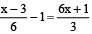2. Solve: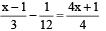3. Solve: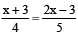4. Solve: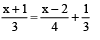5. Solve: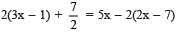1. x = 1

2. x = –1

3. x = 9

4. x = –6

5. x = 5/2

## Mathematics (Maths) Class 8

217 videos|147 docs|48 tests

,

,

,

,

,

,

,

,

,

,

,

,

,

,

,

,

,

,

,

,

,

;